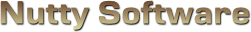Sorry, it appears you don't have support for WebGL.

In order to run this demo, you must meet the following requirements.

• You have a WebGL compatible video card with the latest drivers.
• Your video card is not blacklisted. You can check the current blacklist on Khronos.

Some browsers may require additional configuration in order to get WebGL to run. If you are having problems running this demo, visit the following sites.

Use mouse.

#### Presets

							
/// <summary>
/// </summary>

/// <summary>
/// Material source structure.
/// <summary>
struct MaterialSource
{
vec3 Ambient;
vec4 Diffuse;
vec3 Specular;
float Shininess;
vec2 TextureOffset;
vec2 TextureScale;
};

/// <summary>
/// Attributes.
/// <summary>
attribute vec3 Vertex;
attribute vec2 Uv;
attribute vec3 Normal;

/// <summary>
/// Uniform variables.
/// <summary>
uniform mat4 ProjectionMatrix;
uniform mat4 ViewMatrix;
uniform mat4 ModelMatrix;
uniform vec3 ModelScale;

uniform MaterialSource Material;

/// <summary>
/// Varying variables.
/// <summary>
varying vec4 vWorldVertex;
varying vec3 vWorldNormal;
varying vec2 vUv;
varying vec3 vViewVec;

/// <summary>
/// <summary>
void main ()
{
// Transform the vertex
vWorldVertex = ModelMatrix * vec4(Vertex * ModelScale, 1.0);
vec4 viewVertex = ViewMatrix * vWorldVertex;
gl_Position = ProjectionMatrix * viewVertex;

// Setup the UV coordinates
vUv = Material.TextureOffset + (Uv * Material.TextureScale);

// Rotate normal
vWorldNormal = normalize(mat3(ModelMatrix) * Normal);

// Calculate view vector (for specular lighting)
vViewVec = normalize(-viewVertex.xyz);
}


							
/// <summary>
/// Vertex shader for rendering a 2D plane on the screen. The plane should be sized
/// from -1.0 to 1.0 in the x and y axis. This shader can be shared amongst multiple
/// </summary>

/// <summary>
/// Attributes.
/// <summary>
attribute vec3 Vertex;
attribute vec2 Uv;

/// <summary>
/// Uniform variables.
/// <summary>
uniform mat4 ProjectionMatrix;
uniform mat4 ViewMatrix;
uniform mat4 ModelMatrix;
uniform vec3 ModelScale;

/// <summary>
/// Varying variables.
/// <summary>
varying vec2 vUv;

/// <summary>
/// <summary>
void main ()
{
vec4 worldVertex = ModelMatrix * vec4(Vertex * ModelScale, 1.0);
vec4 viewVertex = ViewMatrix * worldVertex;
gl_Position = ProjectionMatrix * viewVertex;

vUv = Uv;
}


							
/// <summary>
/// Vertex shader for rendering a cubemapped skybox.
/// </summary>

/// <summary>
/// Attributes.
/// <summary>
attribute vec3 Vertex;
attribute vec3 Normal;

/// <summary>
/// Uniform variables.
/// <summary>
uniform mat4 ProjectionMatrix;
uniform mat4 ViewMatrix;
uniform mat4 ModelMatrix;
uniform vec3 ModelScale;

/// <summary>
/// Varying variables.
/// <summary>
varying vec3 vNormal;

/// <summary>
/// <summary>
void main ()
{
gl_Position = ProjectionMatrix * ViewMatrix * ModelMatrix * vec4(Vertex * ModelScale, 1.0);
vNormal = Normal;
}


							
/// <summary>
/// </summary>

#ifdef GL_ES
precision highp float;
#endif

/// <summary>
/// Light source structure.
/// <summary>
struct LightSource
{
vec3 Position;
vec3 Attenuation;
vec3 Direction;
vec3 Colour;
float OuterCutoff;
float InnerCutoff;
float Exponent;
};

/// <summary>
/// Material source structure.
/// <summary>
struct MaterialSource
{
vec3 Ambient;
vec4 Diffuse;
vec3 Specular;
float Shininess;
vec2 TextureOffset;
vec2 TextureScale;
};

/// <summary>
/// Uniform variables.
/// <summary>
uniform int NumLight;
uniform LightSource Light;
uniform MaterialSource Material;
uniform sampler2D Sample0;

/// <summary>
/// Varying variables.
/// <summary>
varying vec4 vWorldVertex;
varying vec3 vWorldNormal;
varying vec2 vUv;
varying vec3 vViewVec;

/// <summary>
/// <summary>
void main ()
{
// vWorldNormal is interpolated when passed into the fragment shader.
// We need to renormalize the vector so that it stays at unit length.
vec3 normal = normalize(vWorldNormal);

vec3 colour = Material.Ambient;
for (int i = 0; i < 4; ++i)
{
if ( i >= NumLight )
break;

// Calculate diffuse term
vec3 lightVec = normalize(Light[i].Position - vWorldVertex.xyz);
float l = dot(normal, lightVec);
if ( l > 0.0 )
{
// Calculate spotlight effect
float spotlight = 1.0;
if ( (Light[i].Direction.x != 0.0) || (Light[i].Direction.y != 0.0) || (Light[i].Direction.z != 0.0) )
{
spotlight = max(-dot(lightVec, Light[i].Direction), 0.0);
float spotlightFade = clamp((Light[i].OuterCutoff - spotlight) / (Light[i].OuterCutoff - Light[i].InnerCutoff), 0.0, 1.0);
spotlight = pow(spotlight * spotlightFade, Light[i].Exponent);
}

// Calculate specular term
vec3 r = -normalize(reflect(lightVec, normal));
float s = pow(max(dot(r, vViewVec), 0.0), Material.Shininess);

// Calculate attenuation factor
float d = distance(vWorldVertex.xyz, Light[i].Position);
float a = 1.0 / (Light[i].Attenuation.x + (Light[i].Attenuation.y * d) + (Light[i].Attenuation.z * d * d));

colour += ((Material.Diffuse.xyz * l) + (Material.Specular * s)) * Light[i].Colour * a * spotlight;
}
}

gl_FragColor = clamp(vec4(colour, Material.Diffuse.w), 0.0, 1.0) * texture2D(Sample0, vUv);
}


							
/// <summary>
/// Fragment shader for rendering a cubemapped skybox.
/// </summary>

#ifdef GL_ES
precision highp float;
#endif

/// <summary>
/// Uniform variables.
/// <summary>
uniform samplerCube Sample0;

/// <summary>
/// Varying variables.
/// <summary>
varying vec3 vNormal;

/// <summary>
/// <summary>
void main ()
{
gl_FragColor = textureCube(Sample0, vNormal);
}


							
/// <summary>
/// Shader for tagging pixels that will be affected by the refraction shader.
/// </summary>

#ifdef GL_ES
precision highp float;
#endif

/// <summary>
/// <summary>
void main ()
{
// Simply set the alpha value
gl_FragColor.w = 0.0;
}


							
/// <summary>
/// Shader to refract all pixels with their alpha channel set to 0.
/// </summary>

#ifdef GL_ES
precision highp float;
#endif

/// <summary>
/// Uniform variables.
/// <summary>
uniform vec2 ImageSize;
uniform vec2 TexelSize;
uniform sampler2D Sample0;

/// <summary>
/// Size of the refraction.
/// <summary>
uniform float Amplitude;

/// <summary>
/// Frequency of the refraction.
/// <summary>
uniform float Frequency;

/// <summary>
/// Relative speed (period) of the refraction.
/// <summary>
uniform float Period;

/// <summary>
/// Random number to animate or mix up the refracted results.
/// <summary>
uniform float RandomNumber;

/// <summary>
/// Varying variables.
/// <summary>
varying vec2 vUv;

// Description : Array and textureless GLSL 3D simplex noise function.
//      Author : Ian McEwan, Ashima Arts.
//  Maintainer : ijm
//     Lastmod : 20110822 (ijm)
//               https://github.com/ashima/webgl-noise
vec3 mod289(vec3 x) { return x - floor(x * (1.0 / 289.0)) * 289.0; }
vec4 mod289(vec4 x) { return x - floor(x * (1.0 / 289.0)) * 289.0; }
vec4 permute(vec4 x) { return mod289(((x*34.0)+1.0)*x); }
vec4 taylorInvSqrt(vec4 r) { return 1.79284291400159 - 0.85373472095314 * r; }
float snoise(vec3 v)
{
const vec2  C = vec2(1.0/6.0, 1.0/3.0) ;
const vec4  D = vec4(0.0, 0.5, 1.0, 2.0);

// First corner
vec3 i  = floor(v + dot(v, C.yyy) );
vec3 x0 =   v - i + dot(i, C.xxx) ;

// Other corners
vec3 g = step(x0.yzx, x0.xyz);
vec3 l = 1.0 - g;
vec3 i1 = min( g.xyz, l.zxy );
vec3 i2 = max( g.xyz, l.zxy );

//   x0 = x0 - 0.0 + 0.0 * C.xxx;
//   x1 = x0 - i1  + 1.0 * C.xxx;
//   x2 = x0 - i2  + 2.0 * C.xxx;
//   x3 = x0 - 1.0 + 3.0 * C.xxx;
vec3 x1 = x0 - i1 + C.xxx;
vec3 x2 = x0 - i2 + C.yyy; // 2.0*C.x = 1/3 = C.y
vec3 x3 = x0 - D.yyy;      // -1.0+3.0*C.x = -0.5 = -D.y

// Permutations
i = mod289(i);
vec4 p = permute( permute( permute(
i.z + vec4(0.0, i1.z, i2.z, 1.0 ))
+ i.y + vec4(0.0, i1.y, i2.y, 1.0 ))
+ i.x + vec4(0.0, i1.x, i2.x, 1.0 ));

// Gradients: 7x7 points over a square, mapped onto an octahedron.
// The ring size 17*17 = 289 is close to a multiple of 49 (49*6 = 294)
float n_ = 0.142857142857; // 1.0/7.0
vec3  ns = n_ * D.wyz - D.xzx;

vec4 j = p - 49.0 * floor(p * ns.z * ns.z);  //  mod(p,7*7)

vec4 x_ = floor(j * ns.z);
vec4 y_ = floor(j - 7.0 * x_ );    // mod(j,N)

vec4 x = x_ *ns.x + ns.yyyy;
vec4 y = y_ *ns.x + ns.yyyy;
vec4 h = 1.0 - abs(x) - abs(y);

vec4 b0 = vec4( x.xy, y.xy );
vec4 b1 = vec4( x.zw, y.zw );

//vec4 s0 = vec4(lessThan(b0,0.0))*2.0 - 1.0;
//vec4 s1 = vec4(lessThan(b1,0.0))*2.0 - 1.0;
vec4 s0 = floor(b0)*2.0 + 1.0;
vec4 s1 = floor(b1)*2.0 + 1.0;
vec4 sh = -step(h, vec4(0.0));

vec4 a0 = b0.xzyw + s0.xzyw*sh.xxyy ;
vec4 a1 = b1.xzyw + s1.xzyw*sh.zzww ;

vec3 p0 = vec3(a0.xy,h.x);
vec3 p1 = vec3(a0.zw,h.y);
vec3 p2 = vec3(a1.xy,h.z);
vec3 p3 = vec3(a1.zw,h.w);

vec4 norm = taylorInvSqrt(vec4(dot(p0,p0), dot(p1,p1), dot(p2, p2), dot(p3,p3)));
p0 *= norm.x;
p1 *= norm.y;
p2 *= norm.z;
p3 *= norm.w;

// Mix final noise value
vec4 m = max(0.6 - vec4(dot(x0,x0), dot(x1,x1), dot(x2,x2), dot(x3,x3)), 0.0);
m = m * m;
return 42.0 * dot( m*m, vec4( dot(p0,x0), dot(p1,x1),
dot(p2,x2), dot(p3,x3) ) );
}

/// <summary>
/// Compute the normal using a sobel filter on the adjacent noise pixels.
///
/// Normally you would output the noise to a texture first and then calculate
/// the normals on that texture to improve performance; however everthing is
/// kept in this shader as a single process to help illustrate what's going on.
/// <summary>
/// <returns>A normal vector.</returns>
vec3 GetNormal ()
{
// Get Sobel values
vec2 uv = vUv * Frequency;
float z = RandomNumber * Period;

float tl = snoise(vec3(uv.x - TexelSize.x, uv.y - TexelSize.y, z));
float t = snoise(vec3(uv.x, uv.y - TexelSize.y, z));
float tr = snoise(vec3(uv.x + TexelSize.x, uv.y - TexelSize.y, z));
float l = snoise(vec3(uv.x - TexelSize.x, uv.y, z));
float r = snoise(vec3(uv.x + TexelSize.x, uv.y, z));
float bl = snoise(vec3(uv.x - TexelSize.x, uv.y + TexelSize.y, z));
float b = snoise(vec3(uv.x, uv.y + TexelSize.y, z));
float br = snoise(vec3(uv.x + TexelSize.x, uv.y + TexelSize.y, z));

// Sobel filter
vec3 normal = vec3((-tl - l * 2.0 - bl) + (tr + r * 2.0 + br),
(-tl - t * 2.0 - tr) + (bl + b * 2.0 + br),
1.0 / Amplitude);

// Return normalized vector
return normalize(normal);
}

/// <summary>
/// <summary>
void main ()
{
// Refract only tagged pixels (that is, the alpha channel has been set)
vec2 offset;
if ( texture2D(Sample0, vUv).w == 0.0 )
{
// Method 1: Use noise as the refraction angle.
// Fast and good results for some scenarios.
//const float pi = 3.141592;
//float noise = snoise(vec3((vUv * Frequency), RandomNumber * Period)) * pi;
//offset = vec2(cos(noise), sin(noise)) * Amplitude * TexelSize;

// Method 2: Get the normal from an animating normalmap to use as the refracted vector.
// Slower, but better results.
vec3 normal = GetNormal();
offset = normal.xy;
}

// Use the colour at the specified offset into the texture
gl_FragColor.xyz = texture2D(Sample0, vUv + offset).xyz;
gl_FragColor.w = 1.0;
}


							
/// <summary>
/// Fragment shader to modify brightness, contrast, and gamma of an image.
/// </summary>

#ifdef GL_ES
precision highp float;
#endif

/// <summary>
/// Uniform variables.
/// <summary>
uniform float Brightness;	// 0 is the centre. < 0 = darken, > 1 = brighten
uniform float Contrast;		// 1 is the centre. < 1 = lower contrast, > 1 is raise contrast
uniform float InvGamma;		// Inverse gamma correction applied to the pixel

uniform sampler2D Sample0;	// Colour texture to modify

/// <summary>
/// Varying variables.
/// <summary>
varying vec2 vUv;

/// <summary>
/// <summary>
void main ()
{
// Get the sample
vec4 colour = texture2D(Sample0, vUv);

colour.xyz = colour.xyz + Brightness;

colour.xyz = (colour.xyz - vec3(0.5)) * Contrast + vec3(0.5);

// Clamp result
colour.xyz = clamp(colour.xyz, 0.0, 1.0);

// Apply gamma correction, except for the alpha channel
colour.xyz = pow(colour.xyz, vec3(InvGamma));

// Set fragment
gl_FragColor = colour;
}



# Refraction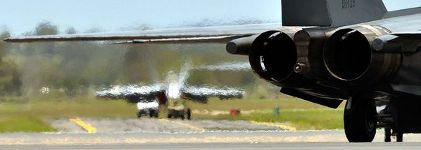Heat haze from an F-111. Photo by Nic MacBean, ABC News

## Introduction

Refraction is the bending of light when passing from one medium into another. When light reaches your eyes, the objects appear bent or distorted in some way. You see this type of physical property whenever you stare at objects within water or when you look off to the horizon on a hot day and see heat haze rising from the ground. This article will cover a refraction technique used to produce these types of effects.

## Refraction

Unlike reflection where a vector bounces off the surface with the same angle, vectors that penetrate transparent objects refract at a different angle, depending on the differences between the two mediums.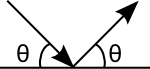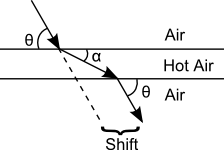Reflection vs Refraction

In the case with heat haze, hot air is less dense then colder air. When light passes through the hot air, it refracts from its trajectory. When it returns to the cooler region, it refracts back to its original angle, but by now the light will have been shifted. To the observer, this makes the object in the distance appear distorted. The hotter the air, the stronger the refractive angle. This is why on a hot summer day the horizon appears to wave in and out smoothly, but when you look at the heat generated from a turbofan on a jet aircraft the distortion is very strong.

## Implementation

The technique demonstrated in the WebGL demo is not physically based. In an ideal implementation, you would cast a ray from the view camera toward the environment and refract the ray as necessary to find an intersection point on an object and colour the pixel. This can be an expensive process. Instead, we will use a rasterization technique. The process is as follows.

1. Create an RGBA framebuffer object.

2. Render the scene to the FBO.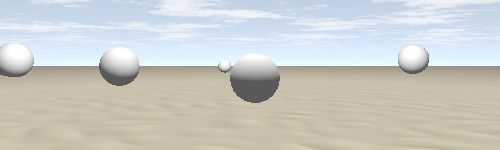3. Render all heat-casting entities to the alpha channel in the FBO. These entities are not actually visible, but by altering the alpha channel the refraction shader will know which pixels to modify. For illustration purposes, the screenshot below displays the refracted area.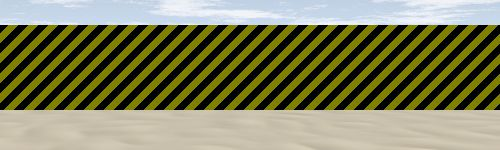4. In the refraction shader, refract the pixels that had their alpha channel modified in step 3. One way to refract the pixels is to use an animating normal map. The demo uses animating 3D simplex noise and a sobel filter to calculate the normalmap. The (X,Y) vector returned by the normal map will point in the direction to acquire the refracted pixel data. The amount you shift is called the amplitude. Small amplitudes represent small changes, like heat haze. Large amplitudes emulate more like water.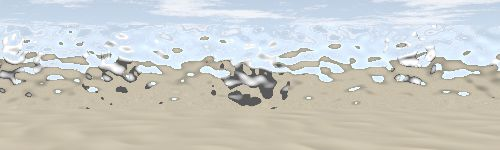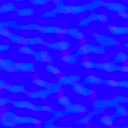Animating normalmap

One last step remains, but is not handled in the demo. Foreground objects will be picked up and incorrectly refracted in the refraction shader. To eliminate this issue, you should render only the objects beyond the refracted surface, pass the texture to the refraction shader, and finally render all foreground objects. This however may not be a trivial solution, especially if you have multiple refracted areas with intersecting polygons. If your amplitudes are within a small range, the rendering defects are not as noticeable and may not even prove to be a quality issue for your game, but it's something to think about.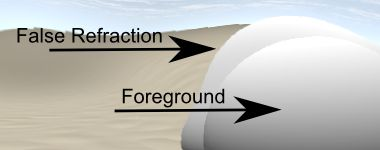The source code for this project is made freely available for download. The ZIP package below contains both the HTML and JavaScript files to replicate this WebGL demo.

The source code utilizes the Nutty Open WebGL Framework, which is an open sourced, simplified version of the closed source Nutty WebGL Framework. It is released under a modified MIT license, so you are free to use if for personal and commercial purposes.